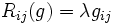# Einstein metric

(diff) ← Older revision | Latest revision (diff) | Newer revision → (diff)

This article defines a property that makes sense for a Riemannian metric over a differential manifold

## Definition

### Symbol-free definition

A Riemannian metric on a differential manifold is said to be an Einstein metric if the Ricci curvature tensor is proportional to the metric tensor.

### Definition with symbols

Let$(M,g)$ be a Riemannian manifold.$g$ is teremd an Eisetin metric if:$R_{ij}(g) = \lambda g_{ij}$

where$\lambda$ is uniform for the whole manifold.

This value of$\lambda$ is termed the cosmological constant for the manifold.

## Relation with other properties

### Stronger properties

The following properties of Riemannian metrics are stronger than the property of being an Einstein metric:

• Ricci-flat metric: This is an Einstein metric with cosmological constant zero, that is, with Ricci curvature zero everywhere
• Constant-curvature metric: This is an Einstein metric with Ricci curvature constant everywhere

### In low dimensions

The following turn out to be true:

• For manifolds of dimension upto three, Einstein metrics are precisely the same as constant-curvature metrics